# MP Board Class 6th Maths Solutions Chapter 13 Symmetry Ex 13.3

## MP Board Class 6th Maths Solutions Chapter 13 Symmetry Ex 13.3

Question 1.
Find the number of lines of symmetry in each of the following shapes. How will you check your answers?
content/uploads/2020/10/MP-Board-Class-6th-Maths-Solutions-Chapter-13-Symmetry-Ex-13.3-1.png” alt=”MP Board Class 6th Maths Solutions Chapter 13 Symmetry Ex 13.3 1″ width=”305″ height=”256″ />
Solution:
(a) Number of lines of symmetry = 4.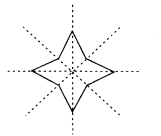(b) Number of lines of symmetry = 1.(c) Number of lines of symmetry = 2.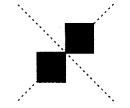(d) Number of lines of symmetry = 2.(e) Number of lines of symmetry = 1.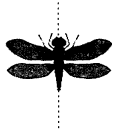(f) Number of lines of symmetry = 2.Question 2.
Copy the following drawing on squared paper. Complete each one of them such that the resulting figure has two dotted lines as two lines of symmetry.Solution: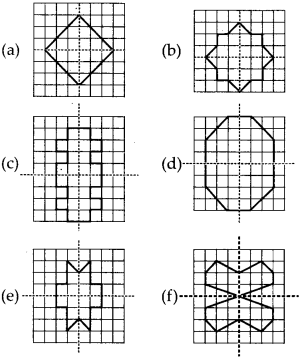Question 3.
In each figure alongside, a letter of the alphabet is shown along with a vertical line. Take the mirror image of the letter in the given line. Find which letters look the same after reflection (i.e. which letters look the same in the image) and which do not. Can you guess why?
Try for O E M N P H L T S V X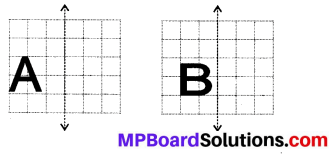Solution: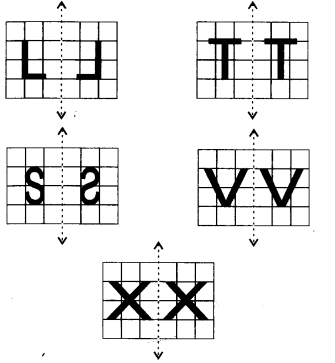Letters which look the same after reflection are A, O, M, H, T, V, X and which do not look the same after reflection are B, E, N, P, L, S.